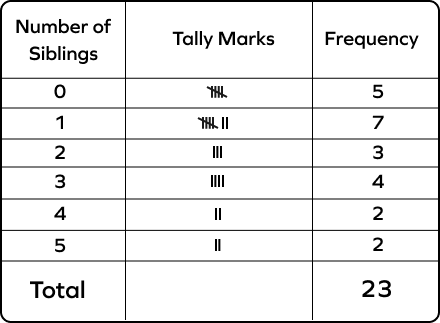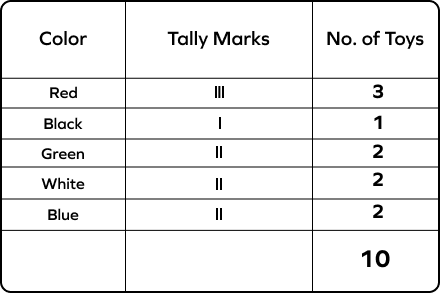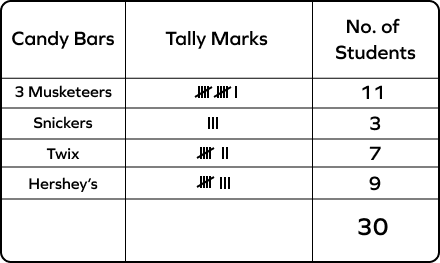# Tally Table – Definition with Examples

## What is a Tally Table?

A tally table is a table that represents the occurrence of an event in a way that calculating it becomes easy.

Suppose your school teacher handed you a list of scores of different students on a quiz and asked you to count how many students got the highest score or how many students got more than a passing score.

What will you do?

In the world of math, you will create a tally table.

Before learning what a tally table is in math,  you need to know what frequency is.

## Frequency

Frequency is the occurrence of an event. The number of times a particular entry is occurring denotes the frequency of that entry.

You need to track this frequency on a tally chart. This track will be recorded in a set of 5 with the help of tally marks.

## Tally Marks

Tally marks are standing and slanted lines. Each line is used to represent each occurrence. They are represented in the set of 5, in which for up to four occurrences of events, straight lines are drawn, and the fifth occurrence of an event is represented as a slanted line over the earlier four lines.

See the table shown below to understand these tally marks better.

## How to Solve a Tally Table?

Let’s say there are 30 students in your class. The given data represents the number of points scored of each student on a quiz:

36  15  30  17  16  15  17  20  30  35  18  27  25  18  30 25  18  30  28  25  35  28  30  28  25   36  35  30  25  36

You have to answer the following questions:

1. How many students got more than 20 points?
2. How many students got the highest number of points?
3. How many students fail the quiz if the passing points are 20?

1. 21 students scored more than 20 points.
2. 3 students scored the highest number of points.
3. 8 students failed the quiz.

A tally table is made to simplify such data.

## Uses of Tally Table Examples in Everyday Life

1. Used in keeping a record of inventories in a store.
2. Used to keep records of payrolls of employees.

## Solved Examples

Example 1. Below are the runs made by a cricket player in 20 balls.

6  1  2  6  5  4  6  2  3  5  4  2  3  4  2  1  4  2  3   1

Make a tally table of the data and answer the following questions.

1. How many times has he scored a “6”?
2. How many times does he score less than 3?
3. How many times has he scored a “4”?

Solution 1.

1. It is clear from the table that the player has scored a “6” 3 times. As there are three lines in the table in front of score 6.
2. He has scored “less than 3” 8 times. Less than 3 means the sum of the frequency of scores 2 and 1, i.e., 3+5.
3. He has scored a “4” 4 times. As the lines in front of score 4 are 4.

1. How many students scored more than 8 points?
2. How many students failed the quiz if the passing score was 4 points?
3. How many students scored the highest number of points?

Solution 2.

1. Two students scored more than 8 points.
2. 7 students failed the quiz. As the passing score is 4 points, the students who failed scored 3, 2, or 1. So, their sum is 7. [1+4+2= 7]
3. Only one student scored the highest number of points.

Example 3: Given below are the ages of 30 people in a building. Make a tally table and answer the following questions:

5  17  30  5  40  17  51  5  62  65  48  23  5  51  17  40  62  65  48  23  30  5  40  30  17  23  39  62  65  48

1. How many people are aged below 18 years?
2. How many people are aged between 20 and 40 years?
3. How many people are over 30 years of age?

Solution 3.

1. Below 18 means 17 and 5. So, there are [5+4] 9 people who are less than 18 years old.
2. Between 20 and 40 means 23, 30, and 39. So, 7 people are between 20 and 40 years of age.
3. Above 30 means 39, 40, 48, 51, 62, and 65. So, 15 people are aged above 30.

## Practice Problems

1

### Read the table and answer the following question. How many people have more than 2 siblings?8
9
5
6
CorrectIncorrect
More than 2 means the sum of the number of people who have 3, 4, and 5 siblings.
4 + 2 + 2 = 8
2

### Read the table and answer the following question. How many people have a red toy?6
9
4
3
CorrectIncorrect
There are three lines in front of “red” in the tally table.
3

### Read the table and answer the following question: How many students have a Snickers bar?11
3
7
9
CorrectIncorrect